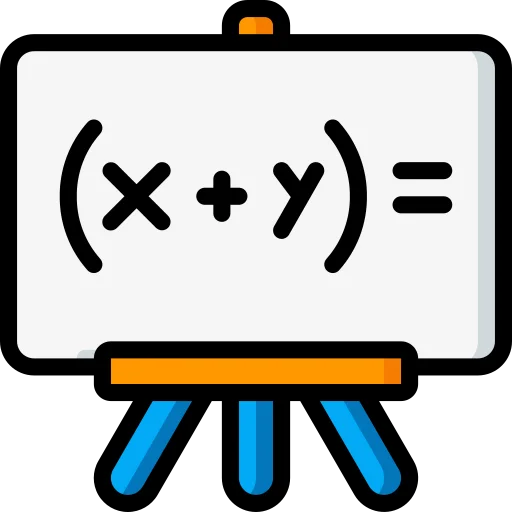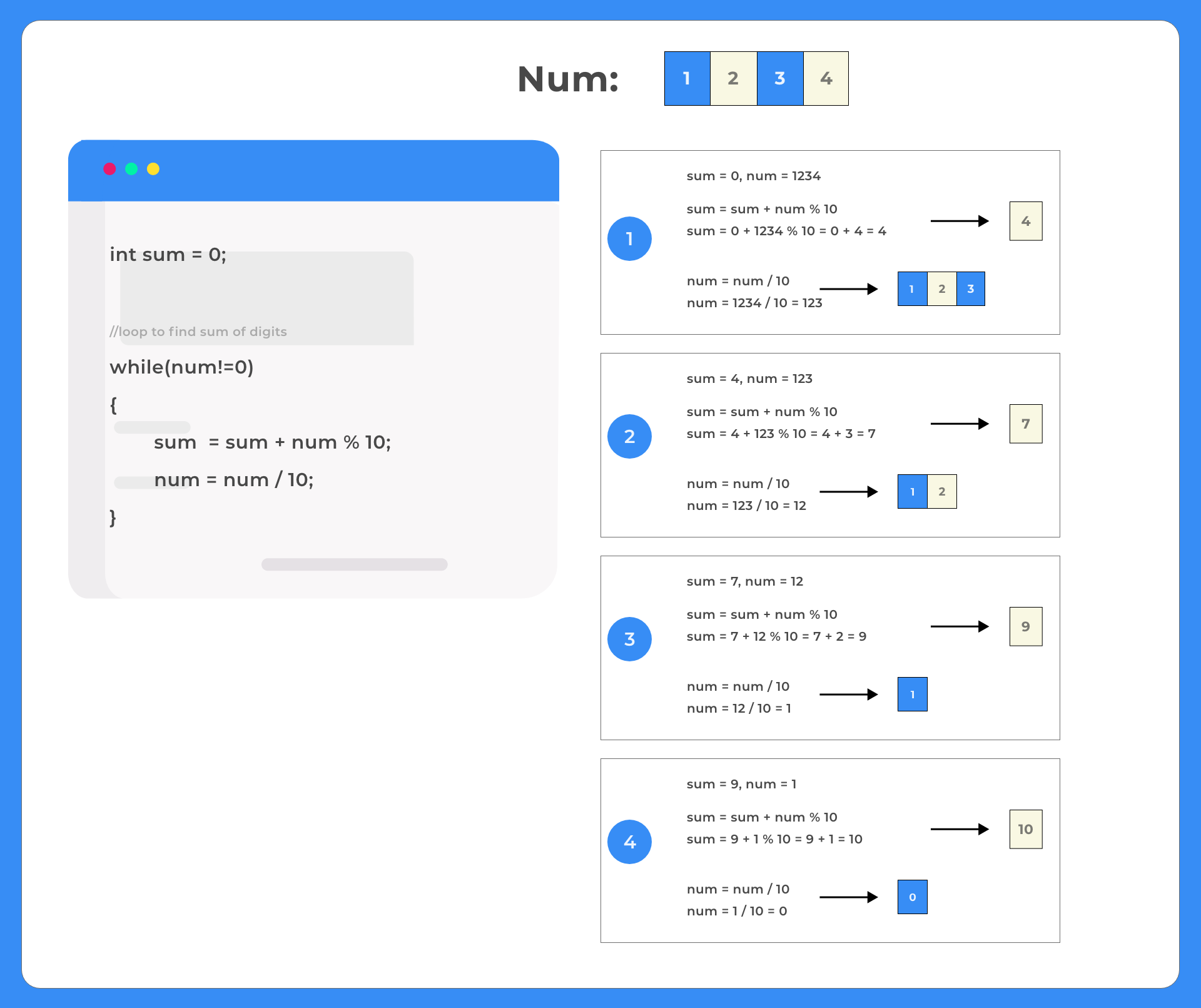# Sum of digits of a Number in C++

## Program to Find the Sum of Digits of a Number in C++

Here we will discuss how to find the sum of digits of a number in C++ programming language.

```We will use loops along with two arithmetic operators:

1. Modulo Operator : '%'
2. Division Operator: '/'

Eg: number = 12345
Sum of digit of number = 1 + 2 + 3 + 4 + 5
Sum = 15```### Algorithm:-

For user input num

• Initialize sum = 0
• While extracting the last digit of num add it to the sum (sum += num % 10)
•  Reduce the number  (num = num / 10
• Keep doing this until num becomes 0### C++ Code

Run

```//C++ Program
//Sum of digits in a number
#include<iostream>
using namespace std;

int main ()
{
int num, sum = 0;

num=1234;
cout <<"\nThe number is: " << num;

//loop to find sum of digits
while(num!=0){
sum += num % 10;
num = num / 10;
}

//output
cout <<"\nSum of digits: " << sum;

return 0;
}
// Time complexity : O(N)
// Space complexity : O(1)
// where N is number of digits in num
```

### Output

```The number is:1234
Sum of digits : 10```

## Method 2

This method uses recursion in c++. Please go through this page if you are new to recursion

### C++ Code

Run

```//C++ Program sum of digits in a number using recursion
#include<iostream>
using namespace std;

int getSum(int num, int sum){

if(num == 0)
return sum;

sum += num % 10;

return getSum(num/10, sum);
}

int main ()
{
int num, sum = 0;
num=12345;
cout <<"\nThe number is:"<<num;

cout <<"\nSum of digits : " << getSum(num, sum);

return 0;
}
// Time complexity : O(N)
// Space complexity : O(1)
// Auxiliary Space complexity : O(N) due to function call stack
// where N is number of digits in num
```

### Output

```The number is:12345
Sum of digits : 15```

## Method 3

This method uses recursion in c++. Please go through this page if you are new to recursion.

This method has a little improvement over the previous recursive method as it doesn’t use extra sum parameter in the recursive function.

### C++ Code

Run

```//C++ Program sum of digits in a number using recursion
#include<iostream>
using namespace std;

int getSum(int num){

if(num == 0)
return 0;

return (num % 10) + getSum(num/10);
}

int main ()
{
int num;
num=12345;
cout <<"\nThe number is:"<<num;

cout <<"\nSum of digits : " << getSum(num);

return 0;
}
// Time complexity : O(N)
// Space complexity : O(1)
// Auxiliary Space complexity : O(N) due to function call stack
// where N is number of digits in num
```

### Output

```The number is:12345
Sum of digits : 15```

## Method 4

Make sure to check ASCII Table here for below method

### Code

Run

```// Time Complexity : O(N)
// Space Complexity : O(1)
#include<iostream>
using namespace std;

int getSum(string num)
{
int sum = 0;

// Traverse through the whole string(char array)
for (int i = 0; i < num.length(); i++)
{
// Ascii value pf numbers start from 48
// subtracting 48 will give us value in int
sum = sum + num[i] - 48;
}
return sum;
}

int main()
{
string num = "9876543219876543219876";

// can also use below
// int len = sizeof(num)/sizeof(num);

cout<< "Sum: " << getSum(num);
return 0;
}```

#### Output

```Sum: 120
```

### Related Banners

Get PrepInsta Prime & get Access to all 200+ courses offered by PrepInsta in One Subscription